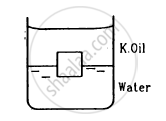Advertisement Remove all ads

# A Cube of Ice Floats Partly in Water and Partly in K.Oil. Find the Ratio of the Volume of Ice Immersed in Water to that in K.Oil. Specific Gravity of K.Oil is 0.8 and that of Ice is 0.9. - Physics

Sum

A cube of ice floats partly in water and partly in K.oil (in the following figure). Find the ratio of the volume of ice immersed in water to that in K.oil. Specific gravity of K.oil is 0.8 and that of ice is 0.9.Advertisement Remove all ads

#### Solution

Given:
Specific gravity of water, $\rho_W$  = 1 gm/cc
Specific gravity of ice, ρice = 0.9 gm/cc
Specific gravity of kerosene oil, ρk = 0.8 gm/cc
Now,
Vice = Vk + Vw
Here,
Vk = Volume of ice inside kerosene oil
Vw = Volume of ice inside water
Vice = Volume of ice
Thus, we have:

$V_{ice} \times \rho_{ice} \times g = V_k \times \rho_k \times g + V_w \times \rho_w \times g$

$\Rightarrow \left( V_k + V_w \right) \times \rho_{ice} = V_k \times \rho_k + V_w \times \rho_w$

$\Rightarrow (0 . 9) V_k + (0 . 9) V_w = (0 . 8) V_k + \left( 1 \right) \times V_w$

$\Rightarrow (0 . 1) V_w = 0 . 1 V_k$

$\Rightarrow \frac{V_w}{V_k} = 1 .$

$\Rightarrow V_w : V_k = 1: 1$

Is there an error in this question or solution?
Advertisement Remove all ads

#### APPEARS IN

HC Verma Class 11, 12 Concepts of Physics 1
Chapter 13 Fluid Mechanics
Q 14 | Page 274
Advertisement Remove all ads

#### Video TutorialsVIEW ALL 

Advertisement Remove all ads
Share
Notifications

View all notifications

Forgot password?